Home > Geometry calculators

 AtoZmath.com - Homework help (with all solution steps) Educational Level Secondary school, High school and College Program Purpose Provide step by step solutions of your problems using online calculators (online solvers) Problem Source Your textbook, etc

 0. Geometry examples Geometry Calculators 1. Coordinate Geometry 2. Graphs 1. Graphs - Plotting of mathematical functions f(x) or f(y) 2. Graph - Using Points and Slope 2.1 Points : (3,-5),(5,3) 2.2 Points on X-axis : -3,5,12,-7,0 2.3 Points on Y-axis : -3,5,12,-7,0 2.4 Lines : x=-3y+5; 2x+y=1; x+2y<=5; x+y>=15 2.5 Line using Slope & point : Slope=7 and Point=(4,6) 2.6 Line using Slope & Y-Intercept : Slope=2 and Y-Intercept=-4 2.7 Line passing through two points : Point1=(3,-5) and Point2=(5,3) 3. Circle 4. Ellipse 5. Parabola 6. Hyperbola 7. Polar Graph 3. Area 1. Circle 2. Semi-Circle 3. RegularHexagon 4. Square 5. Rectangle 6. Parallelogram 7. Rhombus 8. Trapezium 9. Scalene Triangle 10. Right angle Triangle 11. Equilateral Triangle 12. Isosceles Triangle 13. Sector Segment 4. Volume 1. Cuboid 2. Cube 3. Cylinder 4. Cone 5. Sphere 6. Hemi-Sphere 5. Pythagorean Theorem
 1. General Graph 1. y = x 2. y <= sin(x) 3. y >= cos(x) 4. y = sqrt(x) 5. y <= sqrt(x^3-3x) 6. y >= |x| 7. y = x^3-x 8. y <= (e^x-e^(-x))/2 1. x = sin(y) 2. x <= cos(y) 3. x >= sqrt(y) 4. x = sqrt(y^3-3y) 5. x <= |y| 6. x >= sin(y)^3+cos(y)^3 7. x = y+sin(y) 8. x <= (sin(9y)+sin(10y))*sin(0.1y)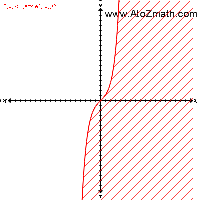2. Graph - Using Points and Slope 1. Points like (3,-5),(5,3) 2. Points on X-axis like -3,5,12,-7,0 3. Points on Y-axis like -3,5,12,-7,0 4. Lines like x=-3y+5; 2x+y=1; x+2y<=5; x+y>=15 5. Line using Slope & point like Slope=7 and Point=(4,6) 6. Line using Slope & Y-Intercept like Slope=2 and Y-Intercept=-4 7. Line passing through two points like Point1=(3,-5) and Point2=(5,3)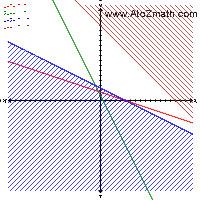3. Circle 1. Circle-1 X^2 + Y^2 = 9 2. Circle-2 (X+1)^2 + Y^2 = 12 3. Circle-3 X^2 + (Y-2)^2 = 15 4. Circle-4 (X+1)^2 + (Y-2)^2 = 9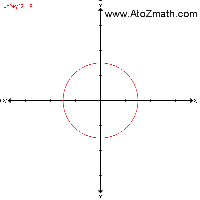4. Ellipse 1. Ellipse-1 X^2/4 + Y^2/9 = 9 2. Ellipse-2 (X+1)^2/4 + Y^2/9 = 12 3. Ellipse-3 X^2/4 + (Y-2)^2/9 = 15 4. Ellipse-4 (X+1)^2/4 + (Y-2)^2/9 = 9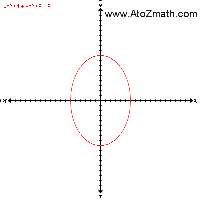5. Parabola 1. Parabola-1 Y = 3X^2 2. Parabola-2 Y = 3X^2 + 1 3. Parabola-3 Y = 3(X+1)^2 4. Parabola-4 Y = 3(X+1)^2 + 1 5. Parabola-5 X = 3Y^2 6. Parabola-6 X = 3Y^2 + 1 7. Parabola-7 X = 3(Y+1)^2 8. Parabola-8 X = 3(Y+1)^2 + 16. Hyperbola 1. Hyperbola-1 X^2/4 - Y^2/9 = 9 2. Hyperbola-2 2. (X+1)^2/4 - Y^2/9 = 12 3. Hyperbola-3 X^2/4 - (Y-2)^2/9 = 15 4. Hyperbola-4 (X+1)^2/4 - (Y-2)^2/9 = 9 5. Hyperbola-5 Y^2/4 - X^2/9 = 9 6. Hyperbola-6 (Y+1)^2/4 - X^2/9 = 12 7. Hyperbola-7 Y^2/4 - (X-2)^2/9 = 15 8. Hyperbola-8 (Y+1)^2/4 - (X-2)^2/9 = 97. Polar Graph 1. R = 4*cos(2*t) 2. R = 4*sin(2*t) 3. R = 2-4*sin(2*t) 4. R = 2-4*cos(2*t) 5. R = 2+4*cos(2*t) 6. R = 2+4*sin(2*t)8. Statistics Graph
1. Histogram
2. Frequency Polygon
3. Frequency Curve
4. Less than type cumulative frequency curve
5. More than type cumulative frequency curve
1. The frequency distribution of the marks obtained by 100 students in a test of Mathematics carrying 50 marks is given below.
Draw Histogram, Frequency Polygon, Frequency Curve, Less than type cumulative frequency curve and More than type cumulative frequency curve of the data.

 Marks obtained 0 - 9 10 - 19 20 - 29 30 - 39 40 - 49 number of students 8 15 20 45 12
 1. Histogram 2. Frequency Polygon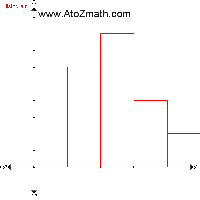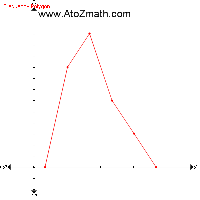3. Frequency Curve 4. Less than type cumulative frequency curve5. More than type cumulative frequency curve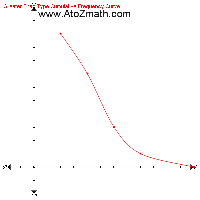1. Circle Area (A) = pi r^2 Circumference (C) = 2 pi r = pi d Diameter (d) = 2 r I know that for a circle Radius = 10 . From this find out Area of the circle. "Here radius " (r)=10 "(Given)""Diameter " (d)= 2 * r = 2 * 10 = 20"Perimeter" = 2 pi r = 2 * 22/7 * 10 = 440/7Area = pi r^2 = 22/7 * (10)^2 = 2200/7
 2. Semi-Circle Area (A) = 1/2 pi r^2 Circumference (C) = pi r = (pi d)/2 Perimeter (P) = pi r + 2 r Diameter (d) = 2 r I know that for a Semi-Circle Radius = 10 . From this find out Area of the Semi-Circle. "Here radius "(r)=10" (Given)""Diameter "(d)= 2 r = 2 * 10 = 20"Circumference" = pi r = 22/7 * 10 = 220/7"Perimeter" = pi r + 2 r = 22/7 * 10 + 2 * 10 = 220/7 + 20 = 360/7"Area" = (pi r^2)/2 = (22/7 * (10)^2)/2 = 1100/7

 3. RegularHexagon Perimeter (P) = 6 a Area (A) = sqrt(3)/4 xx 6 xx a^2 I know that for a Regular Hexagon Side = 10 . From this find out Area of the Regular Hexagon. "Here Side "(a)=10" (Given)""Perimeter" = 6 a = 6 * 10 = 60"Area" = sqrt(3)/4 * 6 * a^2 = sqrt(3)/4 * 6 * 10^2 = 259.8076
 4. Square Diagonal (d) = sqrt(2) a Perimeter (P) = 4a Area (A) = a^2 = d^2/2 I know that for a square Side(a) = 10 . From this find out Area of the square. "Here a" = 10" (Given)""Diagonal" = sqrt(2) * "a" = sqrt(2) * 10 = 14.1421"Perimeter" = 4 * "a" = 4 * 10 = 40"Area" = "a"^2 = 10^2 = 100

 5. Rectangle Diagonal (d) = sqrt(l^2 + b^2) Perimeter (P) = 2(l+b) Area (A) = l b I know that for a rectangle Length = 5 and Breadth = 12 . From this find out Area of the rectangle. "Here one-Side " (l) = 5" and other-Side " (b) = 12" (Given)""Diagonal" = sqrt(l^2 + b^2) = sqrt(5^2 + 12^2) = sqrt(25 + 144) = sqrt(169) = 13"Perimeter" = 2 * "(sum of Sides)" = 2 * (5 + 12) = 34"Area = Product of Sides" = 5 * 12 = 60
 6. Parallelogram Area (A) = ah Perimeter (P) = 2a + 2b I know that for a parallelogram a = 9 , b = 22 and h = 14 . From this find out Area of the parallelogram. "Here " a=9, b=22, h=14" (Given)""Perimeter" = 2 * (a + b) = 2 * (9 + 22)  = 62"Area" = a * h = 9 * 14 = 126

 7. Rhombus Radius (r_1) = (d_1)/2 Radius (r_2) = (d_2)/2 Side (a) = sqrt(r_1^2 + r_2^2) Perimeter (P) = 4 a Area (SA) = (d_1 d_2)/2 I know that for a rhombus d1 = 10 and d2 = 24 . From this find out Area of the rhombus. "Here, we have " d_1 = 10" and " d_2 = 24" (Given)"a^2 = (d_1/2)^2 + (d_2/2)^2a^2 = (10/2)^2 + (24/2)^2a^2 = (5)^2 + (12)^2a^2 = 169a = 13"Perimeter" = 4 * a = 4 * 13 = 52"Area" = 1/2 " (Product of diagonals)" = 1/2 * d_1 * d_2 = 1/2 * 10 * 24 = 120
 8. Trapezium Area (A) = h/2 (a + b) Perimeter (P) = a + b + c + d I know that for a trapezium a = 22 , b = 18 , c = 16 , d = 16 and h = 4 . From this find out Area of the trapezium. "Here " a=22, b=18, c=16, d=16, h=4" (Given)""Perimeter" = a + b + c + d = 22 + 18 + 16 + 16  = 72"Area" = (a + b) * h/2 = (22 + 18) * 4/2 = 80

 9. Scalene Triangle Perimeter (P) = a+b+c S = P/2 = (a+b+c)/2 Area (A) = sqrt(S (S - a) (S - b) (S - c)) I know that for a scalene Triangles a = 3 , b = 4 and c = 5 . From this find out Area of the scalene Triangles. "Here " a=3, b=4, c=5" (Given)""We know that,""Perimeter" = a + b + c = 3 + 4 + 5 = 12 "Semi-Perimeter" = s = (a + b + c)/2 = 12/2 = 6"Here " a=3, b=4, c=5" and semi-Perimeter" = 6"We know that,""Area" = sqrt(s (s - a) (s - b) (s - c)) = sqrt(6 (6 - 3) (6 - 4) (6 - 5)) = 6
 10. Right angle Triangle Diagonal (d) = sqrt(a^2 + b^2) Perimeter (P) = a+b+c Area (A) = 1/2(a b) I know that for a right angle Triangles AB = 5 and BC = 12 . From this find out Area of the right angle Triangles. "Here one Side" = 5" and other Side" = 12" (Given)""We know that,""In triangle ABC, by Pythagoras' theorem"AC^2 = AB^2 + BC^2AC^2 = 5^2 + 12^2AC^2 = 25 + 144AC^2 = 169AC = 13"Perimeter" = AB + BC + AC = 5 + 12 + 13 = 30 "Here base" = 5" and height = "12 "We know that,""Area" = 1/2 * AB * BC = 1/2 * 5 * 12 = 30

 11. Equilateral Triangle Perimeter (P) = 3 a Area (A) = sqrt(3)/4 a^2 I know that for a equilateral Triangles Side = 6 . From this find out Area of the equilateral Triangles. "Here " a = 6" (Given)""We know that,""Perimeter" = 3 * a = 3 * 6 = 18"We know that,""Area" = sqrt(3)/4 * a^2 = 1.732/4 * 6 * 6 = 15.5885
 12. Isosceles Triangle Height (h) = sqrt(a^2 - b^2/4) Perimeter (P) = 2 a + b Area (A) = (b h)/2 I know that for a isosceles Triangles a = 5 and b = 6 . From this find out Area of the isoceles Triangles. "Here base " (b) = 6" and equal side " (a) = 5" (Given)""We know that,""Perimeter" = (2 * "equal Side") + "third Side" = (2 * a) + b = (2 * 5) + 6 = 16"We know that,""Area" = 1/2 * "base" * "height" = 1/2 * b * (sqrt(a^2 - b^2))/4 = 1/2 * 6 * sqrt(5^2 - 6^2)/4 = 1/2 * 6 * 4  = 12

 13. Sector Segment Length of the arc = l = (pi r theta)/180 Area of a minor sector = (pi r^2 theta)/360 I know that for a sector & segment Radius = 10 and angle of measure = 180 . From this find out length of arc of the sector & segment. "Here "r = 10" and " theta = 180" (Given)""Length of the arc " = l = (pi r theta)/180=(22/7 * 10 * 180)/180=31.4286"Area of a minor sector "= (pi r^2 theta)/360=(22/7 * 10^2 * 180)/360=157.1429
 1. Cuboid Diagonal (d) = sqrt(l^2+ b^2+ h^2) Surface Area (SA) = 2 (lb + bh + hl) Volume (V) = lbh I know that for a cuboid Length = 3 , Breadth = 4 , and Height = 5 . From this find out Volume of the cuboid. "Here, we have " l = 3, b=4, h=5" (Given)""Diagonal"^2 = l^2 + b^2 + h^2"Diagonal"^2 = 3^2 + 4^2 + 5^2"Diagonal"^2 = 50Diagonal = 7.0711"Here, we have " l = 3, b=4, h=5" (Given)""Volume" = l * b * h = 3 * 4 * 5 = 60"Here, we have " l = 3, b=4, h=5" (Given)""Total Surface Area" = 2 (lb + bh + lh) = 2 * (3 * 4 + 4 * 5 + 3 * 5) = 2 * (47) = 94"Here, we have " l = 3, b=4, h=5" (Given)""Curved Surface Area" = 2h (l + b) = 2 * 5 (3 + 4) = 70
 2. Cube diameter (d) = sqrt(2) l Diagonal = sqrt(3) l Surface Area (SA) = 6 l^2 Volume (V) = l^3 I know that for a cube Length = 3 . From this find out Volume of the cube. "Here, we have " l = 3"Diagonal" = sqrt(3) l = sqrt(3) * 3 = 5.1962"Here, we have " l = 3"Volume" = l^3 = 3^3 = 27"Here, we have " l = 3"Total Surface Area" = 6 l^2 = 6 * 3^2 = 6 * 9 = 54"Here, we have " l = 3"Curved Surface Area" = 4 l^2 = 4 * 3^2 = 4 * 9 = 36

 3. Cylinder Curved Surface Area (CSA) = 2 pi r h Total Surface Area (TSA) = 2 pi r (r + h) Volume (V) = pi r^2 h I know that for a cylinder Radius = 3 and Height = 10 . From this find out Curved Surface Area of the cylinder. "Here, we have Radius "(r) = 3" and Height "(h) = 10" (Given)""Volume" = pi r^2 h = pi * 3^2 * 10 = 282.7433"Total Surface Area" = 2 pi r (r+h) = 2 * pi * 3 * (3 + 10) = 245.0442"Curved Surface Area" = 2 pi r h = 2 * pi * 3 * 10 = 188.4956
 4. Cone Height (h) = sqrt(l^2 - r^2) Curved Surface Area (CSA) = pi r l Total Surface Area (TSA) = pi r (l + r) Volume (V) = (pi r^2 h)/3 I know that for a cone Radius = 3 and Length = 5 . From this find out Volume of the cone. "Here, we have Radius "(r) = 3" and Slant Height " (l) = 5" (Given)"l^2 = r^2 + h^2h^2 = l^2 - r^2h^2 = 5^2 - 3^2h^2 = 16h = 4"Volume" = (pi r^2 h)/3 = (pi * 3^2 * 4)/3 = 37.6991"Total Surface Area" = pi r (l + r) = pi * 3 (5 + 3) = 75.3982"Curved Surface Area" = pi r l = pi * 3 * 5 = 47.1239

 5. Sphere Surface Area (SA) = 4 pi r^2 Volume (V) = 4/3 pi r^3 diameter (d) = 2r I know that for a sphere Radius = 3 . From this find out Volume of the sphere. "Here, we have Radius"(r) = 3" (Given)""Volume" = 4/3 pi r^3 = 4/3 * pi * 3^3 = 84.823"Total Surface Area" = 4 pi r^2 = 4 * pi * 3^2 = 113.0973"Curved Surface Area" = 4 pi r^2 = 4 * pi * 3^2 = 113.0973
 6. Hemi-Sphere Curved Surface Area (CSA) = 2 pi r^2 Total Surface Area (TSA) = 3 pi r^2 Volume (V) = 2/3 pi r^3 diameter (d) = 2r I know that for a Hemi-Sphere Radius = 3 . From this find out Volume of the Hemi-Sphere. "Here, we have Radius " (r) = 3" (Given)""Volume" = 2/3 pi r^3 = 2/3 * pi * 3^3 = 56.5487"Total Surface Area" = 3 pi r^2 = 3 * pi * 3^2 = 84.823"Curved Surface Area" = 2 pi r^2 = 2 * pi * 3^2 = 56.5487

 5. Pythagoras Theorem Pythagorean Theorem : In a right angled triangle, the square of the length of the hypotenuse is equal to the sum of the squares of the lengths of the remaining sides.i.e. AC^2 = AB^2 + BC^2 Using Pythagoras Theorem, Find out AC when AB = 5 and BC = 12 Here AB=5 and BC=12 (Given) We know that, In triangle ABC, by Pythagoras' theorem AC^2 = AB^2 + BC^2 AC^2 = 5^2 + 12^2 AC^2 = 25 + 144 AC^2 = 169 AC = 13

Dear user,